Courses

# Zero and First order Reactions Class 12 Notes | EduRev

## Chemistry Class 12

Created by: Mohit Rajpoot

## Class 12 : Zero and First order Reactions Class 12 Notes | EduRev

The document Zero and First order Reactions Class 12 Notes | EduRev is a part of the Class 12 Course Chemistry Class 12.
All you need of Class 12 at this link: Class 12

Zero Order Reactions :

The rate law for zero order reactions (n = 0) is written as :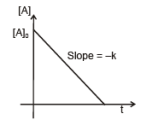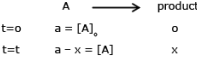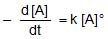-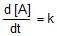-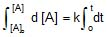[A]o - [A] = kt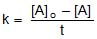=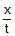Half life (t 1/2) :

Time in which half of initial amount is left.

[A]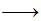[A]o/2

t = o            t = t1/2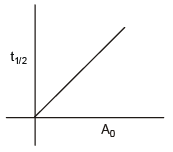k =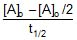t1/2 =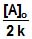Thus, for a Zero order reaction, half life is directly proportional to initial concentration of the reactant.

Clearly, zero order reactions are those, whose rates are not affected by change in concentrations of reactants (i.e., independent of concentration). The rates of such reactions only depend upon temperature. Most of photochemical reactions are zero order reactions. Other examples are : decomposition of HI over the surface of gold and NH3 over tungsten.

Example :

(1)  Photochemical Reactions, Photosynthesis

(2)  CH4 Cl2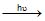Cl - ClCl

CH4 + ClCH3Cl + H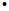First Order reaction

A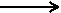product

t = o   [A]o                      -

t = t    [A]                  [P]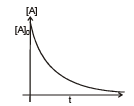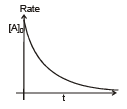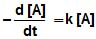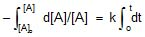ln [A]o/[A] = kt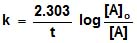Half life (t1/2) :

t = t1/2  [A] = [A]o/2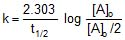=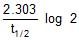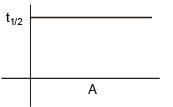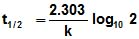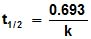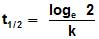[A] =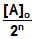where , n = number of half lifes.

Average life :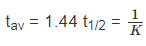Features of a First Order Reaction :

1. A first order reaction must follow above form of rate law for all time instants.

This means if we are given value of A0 and values of x at different time instants [i.e.(A0 - x) as value of reactants after t], the values of k can be calculated for different time instants by using the above first order law.

If the reaction for which the data were given is a first order reaction, then all values of k will approximately equal to each other.

2. The time for half reaction for a first order reaction is independent of initial concentration of reactants.

3. The concentration of reactants in a first order reaction decreases exponentially with time (see figure)

Note that plot of log10 A vs t is linear.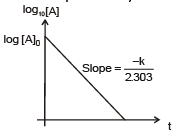Example :

(2) PCl5(g) -> PCl3(g) Cl2(g)

(3) H2O2 → H2O + 1/2O2

(4) NH4NO2 → N2 +2H2O

Rate constant of a first order reaction can also be calculated by measuring the concentration of the reactants at two time instants (if the initial concentration is not known).

If A1 and A2 are the reactant's concentrations at two time instants 't1' and 't2' respectively, then we have :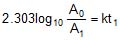...(iii)

and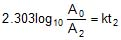... (iv)

Subtracting (iv) from (iii), we get :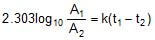Thus, k can be evaluated.

Problem : 4

For a reaction 2NO(g) + 2H2(g) → N2(g) + 2H2O (g) ; the following data were obtained.

 [NO] (mol/L) [H2](mol/L) Rate (mol/L/s) 1. 5 X 10-3 2.5 X 10-3 3 X 10-5 2. 15 X 10-3 2.5 X 10-3 9 X 10-5 3. 15 X 10-3 10 X 10-3 3.6 X 10-4

(a) Calculate the order of reaction.

(b) Find the rate constant.

(c) Find the initial rate if [NO] = [H2] = 8.0 x 10-3 M

Assuming rate law can be expressed as follows :

rate = k[NO]x [H2]y

By analyzing the data :

From observation 1 and 2, we see that [H2] is constant and when [NO] is tripled, the rate is also tripled.

⇒ rate (r) ∝ [NO] ⇒ x = 1

From observations 2 and 3, we see that [NO] is constant; when [H2] is increased four times, the rate also increases four times :

rate ∝ [H2] ⇒ y = 1

⇒ r = k [NO] [H2O]

⇒ The order of reaction w.r.t No and H2 is 1 and the overall order of reaction is 1 1 = 2.

Initial rate = k[NO][H2] = 2.4 x (8 x 10-3)2 = 1.536 x 10-4 mol/L/s.

Second Order kinetics

Case I :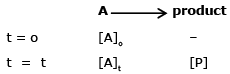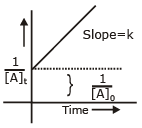-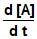= k[A]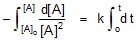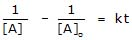k =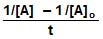Half-life (t1/2) :   [A]t =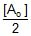, k = 2/[A]o - 1/[A]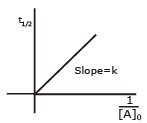t1/2 =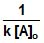Example :

(1) Alkaline hydrolysis of esters.

(2) Self Cannizzaro's reactions,

Case (II):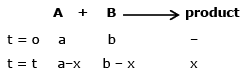-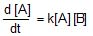,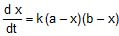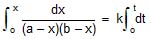,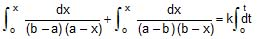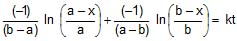ln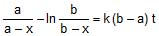k =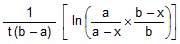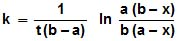Offer running on EduRev: Apply code STAYHOME200 to get INR 200 off on our premium plan EduRev Infinity!

,

,

,

,

,

,

,

,

,

,

,

,

,

,

,

,

,

,

,

,

,

;